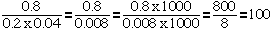Hi my name is Sabrina I am home schooled and I was wondering if you can answer a few questions for me I am a student in 5th grade. I really need the answers to these problems. ok here it goes. [What is the greatest common factor of 20,28, and 36?] next problem is [5k+7(k+8)= ] next problem [Curtis simplified 0.8 divided by 0.2 x 0.04 and got 10. Latoya Simplified and got 1.6. is either correct? Can you explaine how you got the answer please.] Hi Sabrina, What is the greatest common factor of 20,28, and 36? Write the prime factorization of each number. 20 = 225 28 = 227 36 = 2232 From this you can see that 22 divides each of the numbers and none of the other primes divide all three. Thus the greatest common factor is 22 = 4. 5k+7(k+8)= Here you need to use the distributive law to write 7(k+8) as 7k + 78. Thus 5k+7(k+8) = 5k + 7k + 78 = 12k + 56 Curtis simplified 0.8 divided by 0.2 x 0.04 and got 10. Latoya Simplified and got 1.6. is either correct? As I see this problem you are to simplify 0.8 divided by 0.20.04. I would first expand the denominator 0.20.04 = 0.008 To express the fraction 0.8 over 0.008 as an integer over an integer, you need to multiply the denominator by 1000. Thus you also need to multiply the numerator by 1000. Thus I getCheers, Penny Go to Math Central To return to the previous page use your browser's back button.